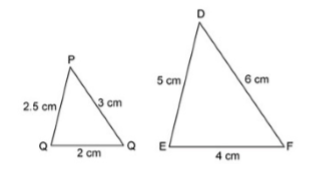# CAT Questions on Similarity of Triangles

0
1358

## CAT Questions on Similarity of Triangles

Download important Similarity of triangle Questions for CAT PDF based on previously asked questions in CAT exam. Practice Similarity of triangle Questions PDF for CAT exam.

Question 1: In two similar triangles ABC and MNP, if AB = 2.25 cm, MP = 4.5 cm and PN = 7.5 cm and m ∠ACB = m ∠MNP and m ∠ABC = m ∠MPN, then the length of side BC , in cm, is

a) 4.5

b) 3.75

c) 4.75

d) 3.5

Question 2: The perimeters of two similar triangles ΔABC andCare 36cm and 24 cm respectively. If PQ = 10 cm, then AB is:

a) 25 cm

b) 10 cm

c) 15 cm

d) 20 cm

Question 3: Δ ABC and Δ DEF are similar triangles. Length of AB is 10 cm and length of the corresponding side DE is 6 cm. What is the ratio of Perimeter of ΔABC to ΔDEF?

a) 5:3

b) 3:5

c) 25:9

d) 9:25

Question 4: Triangle ΔXYZ is similar to ΔPQR. If XY:PQ=5:1. If Area of ΔPQR is 5 sq cm, what is the area (in sq cm) of ΔXYZ?

a) 125

b) 120

c) 100

d) 64

Question 5: The perimeter of two similar triangles ABC and PQR are 36 cms and 24 cms respectively. If PQ = 10 cm then the length of AB is

a) 18 cm

b) 12 cm

c) 15 cm

d) 30 cm

Question 6: ∆ABC and ∆DEF are two similar triangles and the perimeter of ∆ABC and ∆DEF are 30 cm and 18 cm respectively. If length of DE = 36 cm, then length of AB is

a) 60 cm

b) 40 cm

c) 45 cm

d) 50 cm

Question 7: Consider the following figure shown below and choose which of the following equation is correct about the similarity of both triangles?

a) △PQR-△EFD

b) △PQR-△DEF

c) △PQR-△FDE

d) △RQP-△DFE

Question 8: Which of the following condition is TRUE about the similarity of triangle ABC and DEF given below?

a) $\angle A=\angle D, \angle B=\angle E, \angle C=\angle F$

b) $\angle A=\angle E, \angle B=\angle D, \angle C=\angle F$

c) $\angle A=\angle F, \angle B=\angle D, \angle C=\angle E$

d) None of these

Question 9: Which of the following options is/are CORRECT about the similarity of the two triangles?

a) The corresponding sides are proportional to each other.

b) The corresponding angles are equal.

c) The corresponding sides may or may not be equal to each other.

d) All option are correct.

Question 10: If area of similar triangles Δ ABC and Δ DEF be 64 sq cm and 121 sq cm and EF = 15.4 cm then BC equals:

a) 8 cm

b) 18 cm

c) 11.2 cm

d) 8.2 cm

Question 11: Triangle $ABC$ is similar to triangle $PQR$ and $AB : PQ = 2 : 3. AD$ is the median to the side $BC$ in triangle $ABC$ and $PS$ is the median to the side $QR$ in triangle $PQR.$ What is the value of $(\frac{BD}{QS})^2$?

a) $\frac{3}{5}$

b) $\frac{4}{9}$

c) $\frac{2}{3}$

d) $\frac{4}{7}$

As we can see, the 2 triangles are similar. Their sides are in the ratio 2:1 (MPN: ABC). Hence, BC will be 7.5/2 = 3.75 cm. Option B is the right answer.

In Similar triangles , corresponding sides are of same proportion.
$\frac{ Perimeter ΔABC }{ Perimeter ΔPQR }=\frac{AB}{PR}$
$\frac{ 36 }{ 24 }=\frac{AB}{10}$
Ab = 15

It is given that ΔAB $\sim$ ΔDEF

Also, length of AB = 10 cm and length of the corresponding side DE = 6 cm

=> Ratio of Perimeter of ΔABC : Perimeter of ΔDEF = Ratio of corresponding sides = AB : DE

= $\frac{10}{6} = \frac{5}{3}$

$\therefore$ The required ratio is 5 : 3

=> Ans – (A)

Given : $\triangle XYZ \sim \triangle PQR$ and XY:PQ=5:1

To find : ar($\triangle$ XYZ) = $x$ = ?

Solution : Ratio of areas of two similar triangles is equal to the ratio of square of the corresponding sides.

=> $\frac{ar(\triangle XYZ)}{ar(\triangle PQR)}=(\frac{XY}{PQ})^2$

=> $\frac{x}{5}=(\frac{5}{1})^2$

=> $\frac{x}{5}=\frac{25}{1}$

=> $x=25\times5=125$ $cm^2$

=> Ans – (A)

It is given that ΔABC $\sim$ ΔPQR

Also, perimeter of ∆ABC and ∆PQR are 36 cm and 24 cm

=> Ratio of Perimeter of ΔABC : Perimeter of ΔPQR = Ratio of corresponding sides = AB : PQ

= $\frac{36}{24} = \frac{AB}{10}$

=> AB = $\frac{3}{2} \times 10=15$ cm

=> Ans – (C)

It is given that ΔABC $\sim$ ΔDEF

Also, perimeter of ∆ABC and ∆DEF are 30 cm and 18 cm

=> Ratio of Perimeter of ΔABC : Perimeter of ΔDEF = Ratio of corresponding sides = AB : DE

= $\frac{30}{18} = \frac{AB}{36}$

=> AB = $\frac{5}{3} \times 36=60$ cm

=> Ans – (A)

If we compare the corresponding sides of two triangles,=> $\frac{PQ}{DE}=\frac{2.5}{5}=\frac{1}{2}$

Similarly, $\frac{QR}{EF}=\frac{PR}{DF}=\frac{1}{2}$

Since, ratio of all corresponding sides is equal, then $\triangle PQR \sim \triangle DEF$

=> Ans – (B)

The ratio of sides in $\triangle$ ABC and $\triangle$ DEF

= $\frac{AB}{DE}=\frac{BC}{EF}=\frac{AC}{DF}$

= $\frac{14}{7}=\frac{8}{4}=\frac{10}{5}=\frac{2}{1}$

Since, the ratio of corresponding sides are equal, => $\triangle$ ABC $\sim$ $\triangle$ DEF

$\therefore$ $\angle A=\angle D, \angle B=\angle E, \angle C=\angle F$

=> Ans – (A)

If two triangles are similar, then the corresponding sides are proportional to each other. Also, the corresponding angles are equal, but the corresponding sides may or may not be equal to each other. Thus, all are correct.

=> Ans – (D)

Area of Δ ABC = 64 sq.cm
Area of Δ DEF = 121 sq.cm
Given that Δ ABC and Δ DEF are similar triangles.
Then, $\dfrac{\text{Area of } \triangle ABC}{\text{Area of } \triangle DEF} = (\dfrac{BC}{EF})^2$

=> $\dfrac{64}{121} = (\dfrac{BC}{15.4})^2$

=> $\dfrac{BC}{15.4} = \dfrac{8}{11}$

=> $BC = \dfrac{8}{11}\times15.4 = 11.2 cm$

$(\frac{BD}{QS})^2$=4/9